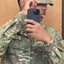MathematicsOpenStudy (bbb911):

Geometry help For questions 5-7, refer to the parallelogram shown . i will post the attachmentsOpenStudy (bbb911):OpenStudy (bbb911):OpenStudy (bbb911):OpenStudy (radar):

Are the opposite sides equal in a parallelogram? so wouldn't side LM = 40 M just like side JKOpenStudy (bbb911):OpenStudy (radar):

If the opposite sides are equal yes. I am going to google parallelogram and verify.OpenStudy (bbb911):

okOpenStudy (radar):

Yes they are equal according to wikipedia. Lets look at the No. 7OpenStudy (bbb911):

okay :)OpenStudy (radar):

Are those two angles at L 56 and 49 degrees, they are blurred and I can't make them out.OpenStudy (bbb911):

on number 5?OpenStudy (radar):

On the drawing of the parallelogram the first one you posted.OpenStudy (bbb911):

yes i did number 5 already but i need 6 and 7 because they go together. so its 56 and 69OpenStudy (radar):

ok i'm thinkingOpenStudy (bbb911):

kk :)OpenStudy (radar):

angle KJM wil equzl angle KLM and that is 56+69 degrees. Isn't that what No. 6 wants?OpenStudy (bbb911):

i think so is that how were find <KJMOpenStudy (radar):

Yes, because opposite angles are equal. Just add 56 and 69 for answer to KJMOpenStudy (bbb911):

and the answer should be 125 degrees rite?OpenStudy (radar):

yes. I am having trble figuring angle JKL. What is the sum of the angles in a parallelogram?OpenStudy (bbb911):

That is were im stupped :(OpenStudy (radar):

OK, the two angles at J and L or 125 degrees each so they add up to 250, now we subtract 250 from the total sum which I believe to be 360 that would leave 110 degrees left for angles JKL plus JML, that would they would be equal to 55 degrees eachOpenStudy (bbb911):

oh i get it now thank you sooo muchOpenStudy (radar):

I am gonna check to see if the sum of all the angles in a parallelogram add up to 360 degrees. Google is my friend.OpenStudy (bbb911):

hehe me 2 :3OpenStudy (radar):

yes, there are 360 degrees in a parallelogramOpenStudy (bbb911):

yay its correct :3OpenStudy (radar):OpenStudy (bbb911):

hehe thank you so much

Latest QuestionsTootie14: Real life poem how do y'all like it I found someone that i think will make me happy but is it worth trusting.
28 minutes ago 6 Replies 3 MedalsAquarytheALPHA: WANNA ZOOM?
1 hour ago 11 Replies 0 Medalsbootyfulgirl: @Supreme01 helppppp
3 hours ago 26 Replies 0 Medalsaxie: How to use bass boosted music on windows! (tutorial)
5 hours ago 11 Replies 2 MedalsCRYSTALC: https://drive.google.com/file/d/1JYWSUWpXf8jVsJChwkz2Y023haObCuy5/view?usp=sharin
3 hours ago 7 Replies 3 MedalsForeverangel: I really need help please. What is the width of the pond, East to West? Round answer to nearest foot.
9 hours ago 1 Reply 0 MedalsForeverangel: I really need help please this is almost due and I can't figure it out. Amplitude
9 hours ago 16 Replies 1 Medal441204: https://www.bandlab.com/2004_diorq/dior-q-loosing-you-prod-billygotitbumpin-44392
6 hours ago 5 Replies 1 Medalalanhernandez: (02.01)Polygon ABCD slides 4 units left and 3 units up on the coordinate plane. I
9 hours ago 1 Reply 0 MedalsForeverangel: Does anyone know a good calculator/website to help me with this question? Find: A
10 hours ago 1 Reply 0 Medals